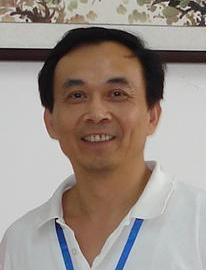陈文雄教授
 发布时间： 2011-01-16   浏览次数： 9102陈文雄，男，博士，河南师范大学特聘教授   电　　话： 0373-3326148   传　　真： 03733326174   通信地址： 数学与信息科学学院   邮　　编： 453007
 个人简历

陈文雄，男，1952年5月生，1986 年中国科学院数学所博士毕业，2006年被聘为河南师范大学特聘教授。

 社会兼职

Communications on Pure and Applied Analysis杂志编辑

 研究方向

 科研成果与奖励

1．美国国家科学基金奖（2000-2004） Nonliear Analysis in Geometry
2．美国国家科学基金奖（2006-2008） Nonlinear Analysis in Convex and Conformal Geometry

 科研项目

1. DMS 0604638, \$123,400, for 2006, 07, and 08; title: `Nonlinear Problems in Convex and Conformal Geometry'.
2. DMS 0072328, \$57,750, for 2000, 01, 02, and 03; title: 'Nonlinear PDEs in Geometric Analysis' .
3. DMS 9704861, \$54,735, for 1997, 98, and 99; title: 'Nonlinear Elliptic Equations in Diferential Geometry'.
4. DMS 9403629, \$24,166, for 1994, 95, and 96; title: 'Nonlinear Elliptic Equations and Systems from Geometry'.
5. DMS 9116949, \$15,109, for 1991; title: 'Methods for Solutions of Some Non- linear Elliptic Equations'.
6. DMS 8722998, for 1987, 88, and 89; title: 'Applications of Analysis to Problems in Geometry'.

 论文著作

1. A sup + inf inequality near R = 0, Advances in Math., 220(2009) 219-245, with Congming Li.Read it.
2. An integral system and the Lane-Emdem conjecture, Disc. Cont. Dyn. Sys., in press, with Congming Li.Read it.
3. Classification of positive solutions for nonlinear differential and integral systems with critical exponents, Acta Mathematica Scientia, in press, with Congming Li.Read it.
4. The Best Constant in Some Weighted Hardy-Littlewood-Sobolev Inequality, Proc. AMS, 136(2008) 955-962, with Congming Li.Read it.
5. A Priori Estimate for the Nirenberg Problem, Disc. Cont. Dyn. Sys. S, 1(2008) 225-233, with Congming Li.Read it.
6. L_p Minkowski problem with not necessarily positive data, Advances in Math, 201 (2006) 77-89. Read it.
7. Classification of solutions for an integral equation, C.P.A.M., 59(2006) 330-343, with Congming Li and Biao Ou.Read it.
8. Qualitative properties of solutions for an integral equation, Disc. Cont. Dyn. Sys., 12(2005), 347-354, with Congming Li and Biao Ou.Read it.
9. Classification of solutions for a system of integral equations, Comm. in PDE, 30(2005), 59-65, with Congming Li and Biao Ou.Read it.
10. Regularity of solutions for a system of integral equations, C.P.A.A. 4(2005), 1-8, with Congming Li.Read it.
11. Weighted system of integral equations, Disc. Cont. Dyn. Sys., special edition (2005) 164-172, with Chao Jin, Congming Li, and Jisun Lim.Read it.
12. On a nonlinear parabolic system-modelling chemical reaction in rivers, C.P.A.A., 4(2005) 889-899, with Congming Li and Eric Wright.Read it.
13. A generalized affine isoperimetric inequality, J. Geom. Anal., 14(2004) 597-612, with Ralph Howard, Erwin Lutwak, Deane Yang, and Gaoyong Zhang.Read it.
14. Moving planes, moving spheres, and a priori estimates, J. of Differential Equations, 195(2003), 1-13, with C.Li.Read it.
15. Gaussian curvature in the negative case, Proceedings of AMS, 131(2003) 741-744, with C.Li.Read it.
16. Prescribing scalar curvature on S^n, Pacific J. Math, Vol. 199, 1(2001), 61-78, with C.Li.Read it.
17. A Note on a Class of Higher Order Conformally Covariant Equations, Disc. Cont. Dyn. Sys., Vol.7, 2(2001), 275-281, with Alice Chang.Read it.
18. Harmonic maps on complete manifolds, Disc. Cont. Dyn. Sys., 4(1999), 799-805, with C.Li.Read it.
19. Some new approaches in prescribing Gaussian and scalar curvature, Proc. of International Confer. on D.S. and D.E.,1998, 148-159, with C.Li.
20. Indefinite elliptic problems with critical exponent, Advances in Nonlinear PDE and Related Areas, World Scientific, 1998, 67-79, with C.Li.Read it.
21. A priori estimates for prescribing scalar curvature equations, Annals of Math. 145(1997) 547-564, with C.Li.Read it.
22. Indefinite Elliptic Problems in a Domain, Disc. Cont. Dyn. Sys., 3(1997), 333-340, with C.Li.Read it.
23. A Note on Kazdan-Warner conditions, J. of Diff. Geom. 41(1995), 259-268, with C.Li.Read it.
24. A necessary and sufficient condition for the Nirenberg's problem, Comm. Pure. & Appl. Math., Vol. XLVIII(1995), 657-667, with C.Li.Read it
25. What kinds of singular metric can admit constant curvature , Duke Math. J., 2(1995) 437-451, with C.Li.Read it.
26. Qualitative Properties of solutions to some nonlinear elliptic equations in R^2, Duke Math. J., 71(1993) 427-439, with C.Li.Read it.
27. A priori estimates for solutions to nonlinear elliptic equations, Arch. Rat. Mech. Anal., 122(1993) 145-157, with C.Li.Read it.
28. Gaussian curvature on singular surfaces, J. Geom. Analysis, 3(1993) 315-334, with C.Li.Read it.
29. Distribution of mass principle and its applications, Proc. of the First World Congress of Nonlinear Analysis, 1993.
30. Classification of solutions of some nonlinear elliptic equations, Duke Math. J., 63(1991) 615-622, with C.Li.Read it.
31. Prescribing Gaussian curvature on surfaces with conical singularities, J. Geom. Analysis, 1(1991) 359-372, with C.Li.Read it.
32. Infinitely many solutions for a nonlinear elliptic equation involving critical Sobolev exponents, Acta Math. Sci., 11(1991) 128-135.
33. A Trudinger inequality on surfaces with conical singularities, Proc. AMS, 108(1990) 821-832.Read it.
34. Scalar curvature on S^n, Math. Annalen, 283(1989) 353-365.Read it.
35. Differential Equations Exam File, Engineering Press, Inc. San Jose, CA.1989, with 26 other professors.
36. A problem concerning the scalar curvature on S^2, Kexue Tongbao (Science Bulletin of China), 33(1988) 533-537, with W.Ding.
37. Scalar curvature on S^2, Trans. AMS, 303(1987) 365-382, with W.Ding.Read it.
38. The existence of multiple solutions for a nonlinear elliptic equation with critical Soblev exponent, Acta Math. Sci., 7(1987) 349-352, with C.Wang.
39. The numerical curvature problem on S^2, Kexue Tongbao, 32(1987) 4-7, with W.Ding.
40. The existence and stability of the Cauchy-Dirichlet problems for nonlinear parabolic equations, J. Hangzhou Univ., 1987, with C.Wang.
41. A non-local boundary value problem for elliptic equations of higher order, J. Hangzhou Univ., 13(1986) 403-408, with C.Wang.
42. The existence of infinitely many solutions for a class of nonlinear elliptic boundary value problems, Annual J. Math., 7A(1986) 154-160, with C.Wang.
43. The existence of infinitely many solutions of non-local boundary value problems for nonlinear elliptic equations, Acta Math. Sci., 5(1985) 223-231,with C.Wang.
44. The existence of solutions for nonlinear Dirichlet problems at resonance, J.Hangzhou Univ., 11(1984) 182-189.
45. A class of boundary value problems for nonlinear elliptic equations, J. Hangzhou Univ., 10(1983) 458-465.